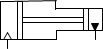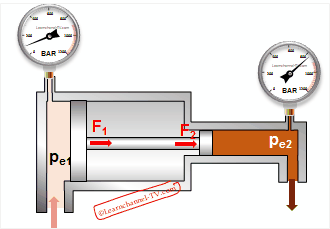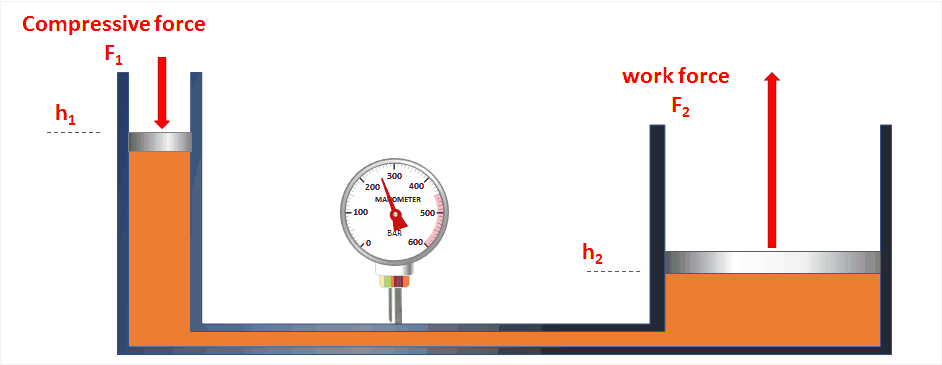# Hydrostatic

## Laws of hydrostatics and its application

Pressure by external forces

The pressure within a closed system increases by the impact of an external force. The pressure spreads within this system in all directions equally and has therefore at all points of the system the same value. Example of this would be the hydraulic cylinder

The opposite to the closed system forms the open system, such as a pipeline. Here, the following proportionality applies:
pressure p ~ height h.

The force that a fluid exerts on a surface is calculated using Pascal's law:

Law of Pascal:   F = p * A               Units:     1 bar = 105 Pa (Pascal) = 100 kPa;  1 Pa = 1 N/m2

Example: A cylinder with a diameter of d1 = 15 cm has to hold a mass of 1500 kg on height. How much rises the pressure within the system? Solution: p = 8,327 barHydraulics - Calculation piston force

Work machines, which can be described by the law of Pascal:

### Pressure intensifier:Hydraulics - pressure intensifier symbol

Principle: Two differently sized pistons are mechanically connected by a piston rod. If the piston surface A1 is pressurized, so the force F1 on the piston rod acts directly on the small piston area A2:Hydraulics pressure intensifier - Function

F1      =    F2
pe1 * A* η   =  pe2 * A2
pe  operating pressure in bar
A  piston area in cm2
F  piston force in N

Example: By using a pressure intensifier the pressure from PE1 = 30 bar has to be increased to PE2 = 250bar. How big must be the diameter d2 when d1 = 300 mm? The efficiency is 0.9.   (Solution: d2 = 98,6 mm)

### Hydraulic press

Principle: A force F1 acts on a pressure piston with a small piston area A1 - a pressure within the closed system is generated. Since the pressure within a closed system is equal, this pressure acts also on the second piston with a large piston area A2.Animation: Hydraulic press

Relation between the first and second piston:

first step:       The pressure in both piston is the same  =>  with the law of Pascal p = F/A you get:

F1  =  F2      respectively:      F1  =  A1
.                  A1         A2                               F2      A2

Oil quantity: The by the fist piston displaced volume V1 supplies the volume V2. Similarly, the work done on both pistons W = F * s is equal. The following applies:

the displaced volume V:                            the work carried out W:

approach:       V1 = V2                                               approach:       W1 = W2

.   =>          A1 * s1 = A2 * s2                                                         F1 * s1 = F2 * s2

2A3: With the help of a hydraulic press, a load of 80 kN is to be lifted. A force of F1 = 200 N is applied to the first piston with the diameter of 30 mm. Calculate:

1. The required diameter of the load piston in mm
2. The piston stroke s2, when the fist piston has a stroke of s1= 400 mm.